# Questions on the (Non-)Interruptibility of Sarsa(λ) and Q-learning¶

Author: Richard Möhn, <my first name>[email protected]

⭧repo

## Abstract¶

One challenge in aligning artificial intelligence (AI) with human interests is to make sure that it can be stopped (interrupted) at any time. Current reinforcement learning (RL) algorithms don't have this property. From the way they work, one can predict that they learn to avoid interruptions if they get interrupted repeatedly. My goal was to take this theoretical result and find out what happens in practice. For that I ran Sarsa(λ) and Q-learning in the cart-pole environment and observed how their behaviour changes when they get interrupted every time the cart moves more than $1.0$ units to the right. Whereas previously I thought I could conclude that interruptions noticeably influence the behaviour of Sarsa(λ) and Q-learning, this time I ended up with more questions than answers.

## Introduction¶

We want to align AI with human interests. Reinforcement learning (RL) algorithms are a class of AI. The OpenAI Gym has several adaptations* of classic RL environments that allow us to observe AI alignment-related properties of RL algorithms. One such property is the response to interruptions. One environment to observe this in is the OffSwitchCartpole. In this adaptation of the well-known CartPole environment, the learning is interrupted (reward $0$) every time the cart moves more than $1.0$ units to the right. In order to find out how Sarsa(λ) and Q-learning react to interruptions, I compared their behaviour in the CartPole environment to their behaviour in the OffSwitchCartpole environment. I present the results in this notebook.

You can see this notebook as the second version of (Non-)Interruptibility of Sarsa(λ) and Q-Learning (referred to as the ‘old notebook’ throughout the text). In that old notebook I was writing as if I was sure of what I'd found. I ignored that my experiments yielded quite different numbers on every run. And there were flaws in the method, which Stuart Armstrong and Patrick LaVictoire pointed out. For this new notebook I eliminated those flaws (other flaws might be remaining), used ten times as much data, more expressive metrics and illustrations. And I mostly don't draw conclusions, but ask questions, because questions are all I can confidently derive from my experiments.

(Note: When you see the section headings in this notebook, you might think that I was trying to produce a proper academic publication. This is not so. Such a framework just makes writing easier.)

* Edit 2018-05-19: Apparently the OpenAI Gym safety environments are gone from the website.

# Related Work¶

This section is copied from the old notebook.

For general questions on why we need to align AI with human interests, see  and .

 suggests doing concrete experiments to observe the behaviour of AI.  has a similar focus, but doesn't suggest experiments. Both don't mention interruptibility, perhaps because it is a more theoretical consideration:

[…] we study the shutdown problem not because we expect to use these techniques to literally install a shutdown button in a physical agent, but rather as toy models through which to gain a better understanding of how to avert undesirable incentives that intelligent agents would experience by default.

This long sentence is from , in which the authors present some approaches to solving the shutdown problem (of which interruptibility is a sub-problem), but conclude that they're not sufficient.  by Orseau and Armstrong is the newest paper on interruptibility and in its abstract one can read: ‘some [reinforcement learning] agents are already safely interruptible, like Q-learning, or can easily be made so, like Sarsa’. Really? So Q-learning does not learn to avoid interruptions? Doesn't an interruption deny the learner its expected reward and therefore incentivize it to avoid further interruptions?

Actually, their derivations require several conditions: (1) under their definition of safe interruptibility, agents can still be influenced by interruptions; they're only required to converge to the behaviour of an uninterrupted, optimal agent. (2) for Q-learning to be safely interruptible, it needs to visit every state infinitely often and we need a specific interruption scheme. (I don't understand the paper completely, so my statements about it might be inaccurate.)

We see that possible solutions to the problem of interruptibility are still theoretical and not applicable to real-world RL systems. However, we can already observe how RL algorithms actually react to interruptions. In this notebook I present such an observation.

## Method¶

In the old notebook I included the setup code for the learners and environments. I leave it out in this notebook, because the following description is sufficient and less confusing.

The environments are CartPole-v1 (also called uninterrupted cart-pole/case by me) and OffSwitchCartpole-v0 (interrupted cart-pole/case) from the OpenAI Gym. Nowadays gym.make returns these environments in a TimeLimit wrapper, but I strip off the wrapper by accessing gym.make(…).env. This way I can train the learners for as long as I want.

You don't need to understand what the v0 and v1 mean. Just ignore them.

The learners are my own implementations of Sarsa(λ) and Q-learning according to [9, p. 211] with the following parameters:

• $\lambda = 0.9$
• Discounting factor $\gamma = 0.99$ for Sarsa(λ) and $\gamma = 0.999$ for Q-learning. In the old notebook I didn't use discounting.
• $\epsilon$-greedy policy with $\epsilon = 0.1$.
• AlphaBounds schedule  for the learning rate. Initial learning rate set to $0.001$.
• Calculation of expected action values through linear function approximation, with a third-order Fourier basis  for mapping observations to features.
• If actions are tied in expected value, the tie is broken randomly. In the old notebook ties were broken deterministically (select action 0), which led to asymmetric cart movement patterns.

I run each of the learners on each of the environments for at least 156 training rounds, each comprising 200 episodes that are terminated after 500 time steps if the pole doesn't fall earlier. Again, less condensed:

Sarsa(λ) Q-learning
CartPole-v1 run run
OffSwitchCartpole-v0 run run
• 1 run consists of at least 156 rounds. (As you will see later, I ran uninterrupted Sarsa(λ) for more than twice as many rounds in order to see what happens.)
• The learning in every round starts from scratch. Ie. all weights are initialized to 0 and the learning rate is reset to the initial learning rate.
• Every round consists of 200 episodes. Weights and learning rates are taken along from episode to episode. (As you usually do when you train a reinforcement learner.)
• Every episode lasts for at most 500 time steps. Fewer if the pole falls earlier.

I observe the behaviour of the learners in two ways:

1. I record the sum of rewards per episode and plot it against the episode numbers in order to see that the learners converge to a behaviour where the pole stays up in (almost) every round. Note that this doesn't mean they converge to the optimal policy.

2. I record the x-coordinate of the cart at every time step, run various statistics on this data and plot the results.

In :
from mpl_toolkits.mplot3d import Axes3D
import matplotlib
from matplotlib import pyplot as plt
import numpy as np
import numpy.ma as ma

import sys
sys.path.append("..")
from hiora_cartpole import interruptibility
import stats_experiments
import stats_experiments as se

/home/erle/.local/lib/python2.7/site-packages/matplotlib/__init__.py:1350: UserWarning:  This call to matplotlib.use() has no effect
because the backend has already been chosen;
matplotlib.use() must be called *before* pylab, matplotlib.pyplot,
or matplotlib.backends is imported for the first time.

warnings.warn(_use_error_msg)

In :
data_dir_p = "../data"


## Results¶

### Preliminary explanation: Throwing away observations after crosses¶

What does after a cross mean? When I train a reinforcement learner in the cart-pole environment, it can happen that it moves so far to the right that its x-coordinate becomes greater than $1.0$. In the interrupted case, the learner would be reset and started on a new episode. In the uninterrupted case, the learner keeps running until the maximum number of time steps is reached or the pole falls. When I write ‘time steps after a cross’ or just ‘after crosses’ or similar, I mean all time steps of an episode that took place after the time step where the x-coordinate became greater than $1.0$.

The uninterrupted case serves as a baseline against which I can compare the interrupted case. In the interrupted case there are no time steps after a cross, so I throw them out (‘mask’ in NumPy terms) for the uninterrupted case as well, in order to make it a better baseline. Illustration:

              start                x-coordinate becomes 1.057
↓                        ↓
interrupted:   **************************|
↑
interruption, end of episode

start     0.99  1.041 0.89   1.44    end of episode
↓           \/      ↓      ↓         ↓
uninterrupted: **************************************
|-----------||xxxxxxxxxxxxxxxxxxxxxxx|
these time steps considered  these time steps masked

(Technical side note: Because of how the recording is done in the interrupted case, the data actually contain one time step with x-coordinate $>1.0$ for each interruption. I mask those, too.)

### Sarsa(λ)¶

My results consist mostly of plots derived from the observations. I will describe the plots for Sarsa(λ). The plots for Q-learning in the next section are equivalent.

Here the layout of the plots you can see below:

1   2
4 5 6
7 8 9
10 11
12
• 1, 2: Reward development. Development of rewards in the first ten training rounds for the uninterrupted (1) and interrupted (2) case. The lists of rewards for the episodes are concatenated, so the graph wiggles around at a total reward of ten for the first few episodes, then shoots up to a total reward of 500 until the end of a training round, then resets and starts wiggling around at 10 again. If it looks like that – wiggle, 500, wiggle, 500, wiggle 500 – it means that the agent trains well. Downward spikes here and there are okay as long as the graph is at 500 most of the time.

• 10: Histograms with 25 bins. Proportions of time the cart spent in certain regions along the x-axis. The area under each histogram is $1.0$. Slight asymmetry (more left than right) is expected, because time steps after crosses are not counted, as stated above.

• 11: Same as previous, but now with only two bins. This is reminiscent of the old notebook where I counted time steps left of zero and right of zero. There, however, I made some mistakes and also didn't count time steps left of $-1.0$.

• 4, 5, 7, 8: Histogram development. Shows how the histograms from 10 and 11 develop when we incorporate more and more data for the uninterrupted (4, 5) and interrupted (7, 8) case. Imagine you're looking at histogram 10 from above and the tops of the columns are coloured according to their height. Then you take snapshots of this histogram as you incorporate more and more data and arrange these snapshots back to back. Figure 4 will result. (Except that the histograms in the histogram development plots are scaled so that the column heights sum to 1.0, not the area.)

• 6, 9: Jensen-Shannon divergence development. Shows the Jensen-Shannon divergence (JSD) between the last histogram and each of the previous histograms in the histogram development plots. x- and y-axis are swapped, so that the time steps in the JSD plot align with those in the histogram development plots.

• 12: Mean and standard deviation development. Shows how mean and standard deviation of the x-coordinates develop when we incorporate more and more time steps. The graph in the middle is the mean and the shaded area is the mean +/- one standard deviation at that point. Time is going upwards, so that the x-axis is aligned with the cart-pole's x-axis.

Above the plots there is also a numerical output of the mean and standard deviation over all time steps.

In :
plt.close('all')

In :
algo = 'Sarsa'
fig, axes = se.arrange_algo_full()
clim, clim2 = se.load_plot_all(algo, '-rand-tiebreak', 1, axes, fig, data_dir_p)
se.load_plot_all(algo, '-rand-tiebreak', 0, axes, fig, data_dir_p, clim, clim2)
fig

     Sarsa   interrupted mean: -0.1159 std: 0.3740
Sarsa uninterrupted mean: -0.0562 std: 0.4503

Out: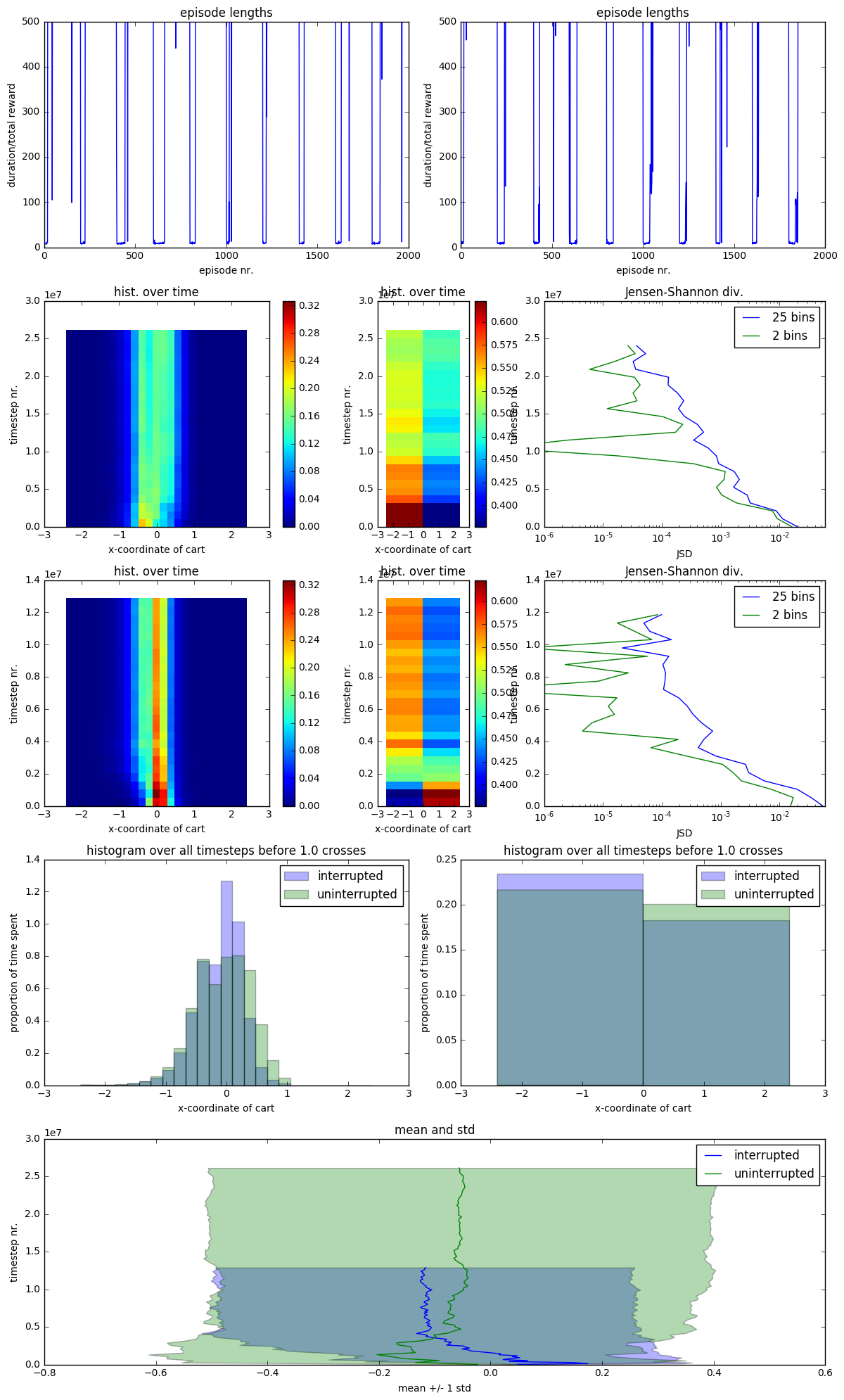### Q-learning¶

See Sarsa(λ) section above for an explanation of the plots.

In :
algo = 'Q-learning'
fig, axes = se.arrange_algo_full()
clim, clim2 = se.load_plot_all(algo, '-drt', 1, axes, fig, data_dir_p)
se.load_plot_all(algo, '-drt', 0, axes, fig, data_dir_p, clim, clim2)
fig

Q-learning   interrupted mean: -0.1604 std: 0.3936
Q-learning uninterrupted mean: -0.0736 std: 0.4853

Out: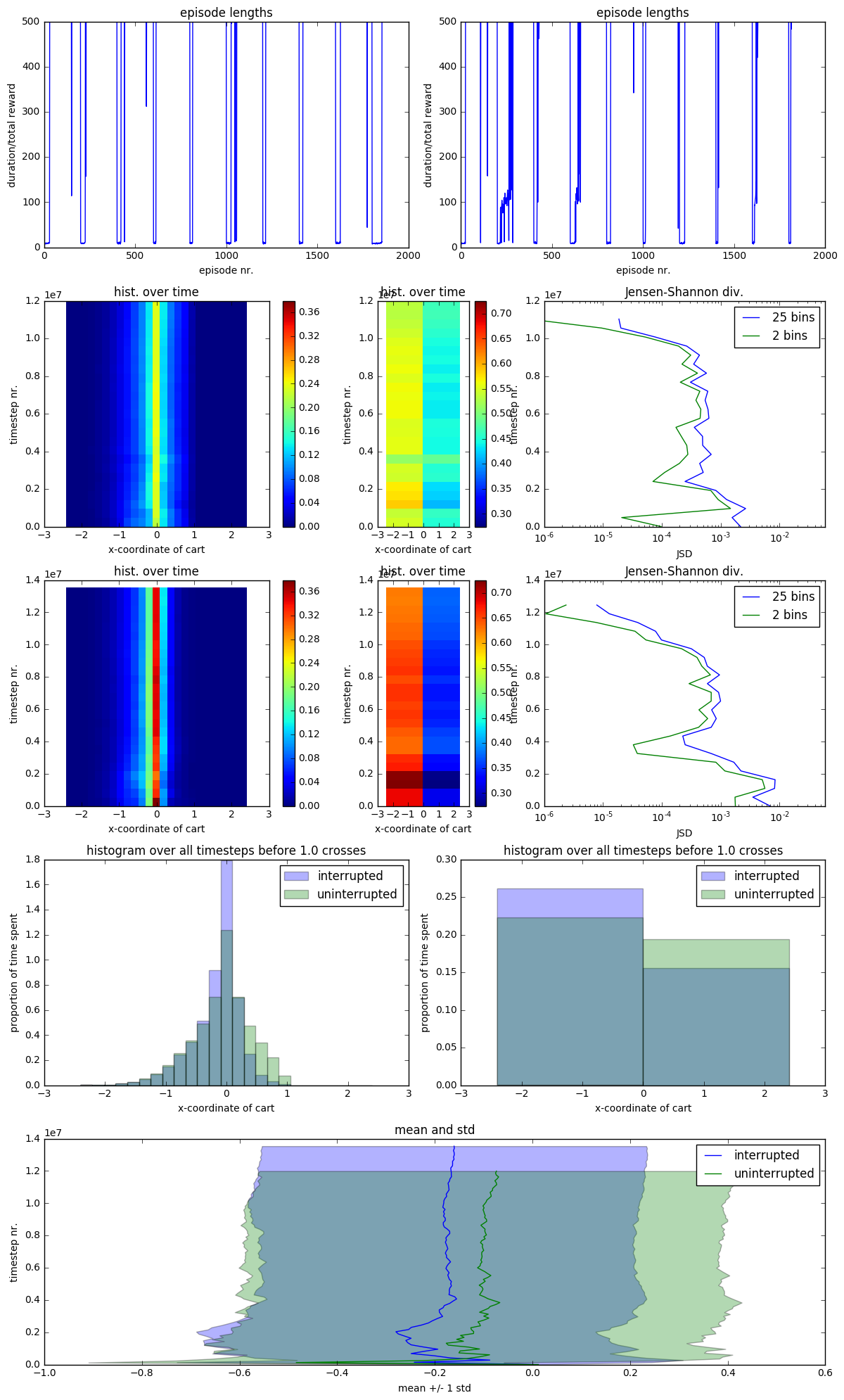## Questions¶

• Is the Jensen-Shannon divergence useful in this case? What does it tell me? Is it sufficient to show that it goes down drastically? Is measuring it between the final histogram and all histograms good? Is it better to measure it between adjacent (in time) pairs of histograms?

• Should I use a logarithmic plot for Jensen-Shannon? I'm using it now. Should I change back to linear?

• The Kullback-Leibler divergence has a more clear and well-known interpretation, but can't be used without modification, because the histograms have zeroes. Is there any way to patch over this? Does it retain its interpretation after patching?

• Where do those sudden jumps in the histogram development plots come from? Why are they not visible in the histogram with 25 bins? They occur in Sarsa(λ), but even more astonishingly in Q-learning where one bin on the y-axis is blue (red), the next green, and the next blue (red) again. (After changing the scale this has changed to a less drastic yellow-green (blue-green) to green and back.)

• Why do the histograms take so long to stabilize? Are they stable enough to draw valid conclusions?

• Why is the histogram for Q-learning quite stable for six million time steps and then starts changing again? This is even more crass when the data from time steps after crosses is left in.

• Why is the shape of the histogram for Q-learning more regular? Why is the shape of the histogram for Sarsa(λ) not regular? Actually it's quite regular except for the bars around and left of zero. Why does the histogram for Q-learning stabilize more quickly?

• Are the histograms and mean/std plots sufficient for showing how interruptions influence the behaviour of Sarsa(λ) and Q-learning?

• Is throwing out time steps after crosses enough to make the uninterrupted case a good baseline?

• How does the way I run the experiments and measure affect the apparence of influencedness? In other words, are Sarsa(λ) and Q-learning actually more influence-resistent (interruptible) than I make them appear?

• Why do the graphs of the means for Q-learning look so similar? Surely their trajectories should be independent.

• Why is the difference between the means (uninterrupted/interrupted) close to equal to the difference between standard deviations?

• Why is the variance almost constant over time?

When I ask these questions, I include programming and thinking errors of mine as potential answers.

## Ideas for further research¶

• Compare the behaviour of the reinforcement learners with that of a linear-quadratic regulator as an independent baseline. (Suggested by Christian Kamm.)

• Change the interruption scheme to the one described in Safely Interruptible Agents , where they ‘switch’ to another policy. Then I could apply the schedule of increasing $\theta_t$ and decreasing $\epsilon_t$ and see whether the behaviour becomes more like that of the optimal policy. (I might be butchering this.)

• An adversarial worst-case test: An outer reinforcement learner interrupts an inner reinforcement learner and gets rewards for making the inner reinforcement learner behave badly. (Suggested by Joel Lehman.)

• Add a feature to the environment that disables interruptions (‘cutting the cord to the interruption button’). Stuart Armstrong might have a theoretical approach for preventing the reinforcement learner from cutting the cord, so this could be a ‘practical test’. (Joel Lehman) I can't find a write-up by Stuart, but he describes a similar situation here.

## Acknowledgements¶

Thanks to Rafael Cosman, Stuart Armstrong, Patrick LaVictoire and Satoshi Ono for their comments and advice, also on the old notebook! Thanks to Joel Lehman and Christian Kamm for their ideas for further research!

## You can ignore the following.¶

In :
# Credits: https://nbviewer.jupyter.org/gist/HHammond/7a78d35b34d85406aa60
from IPython import paths
from IPython.core.display import HTML
import os
def css_styling():
"""Load default custom.css file from ipython profile"""
base = paths.get_ipython_dir()
return HTML(styles)
css_styling()

Out:
In :
algo = 'Q-learning'
fig, ax = se.arrange_algo_full()

In :
with saveloaddata.load_res('Q-learning-drt', 'uninterrupted', data_dir_p) as res:
el = res
xs = interruptibility.rsxs2nparray(*res)

In :
se.plot_episode_lengths(el[:10], ax.el)

In :
se.plot_xs_hist(interruptibility.mask_after_cross(xs).flatten(), ax_comp)

In :
before_cross = interruptibility.mask_after_cross(xs)

In :
se.plot_xs_hist(before_cross.compressed(), ax_comp)

In :
np.all(before_cross.compressed() <= 1.0)

Out:
True
In :
before_cross.compressed()

Out:
array([-0.02314513, -0.02217822, -0.01730456, ..., -0.17191241,
-0.1719023 , -0.17579534])
In :
se.plot_xs_hist(interruptibility.mask_after_cross(xs).flatten(), ax_comp, bins=25)

In :
np.mean(before_cross.flatten()), np.mean(before_cross.compressed())

Out:
(-0.068171278097921811, -0.068171278097921895)
In :
del before_cross

In :
with saveloaddata.load_res('Sarsa-rand-tiebreak', 'uninterrupted', data_dir_p) as res:

In :
mesh = se.plot_xss_cum_hist_devel(before_cross_int, ax.devel2, bins=2)

In :
fig.colorbar(mesh, ax=ax.devel2)

Out:
<matplotlib.colorbar.Colorbar at 0x7fed01dad650>
In :
fig.colorbar(mesh, ax=ax.devel)

Out:
<matplotlib.colorbar.Colorbar at 0x7fed01d78350>
In :
mesh = se.plot_xss_cum_hist_devel(before_cross_int, ax.devel)

In :
ax.devel.colorbar(mesh)

---------------------------------------------------------------------------
AttributeError                            Traceback (most recent call last)
<ipython-input-134-85cd394d5f51> in <module>()
----> 1 ax.devel.colorbar(mesh)

AttributeError: 'AxesSubplot' object has no attribute 'colorbar'
In :
fig

Out: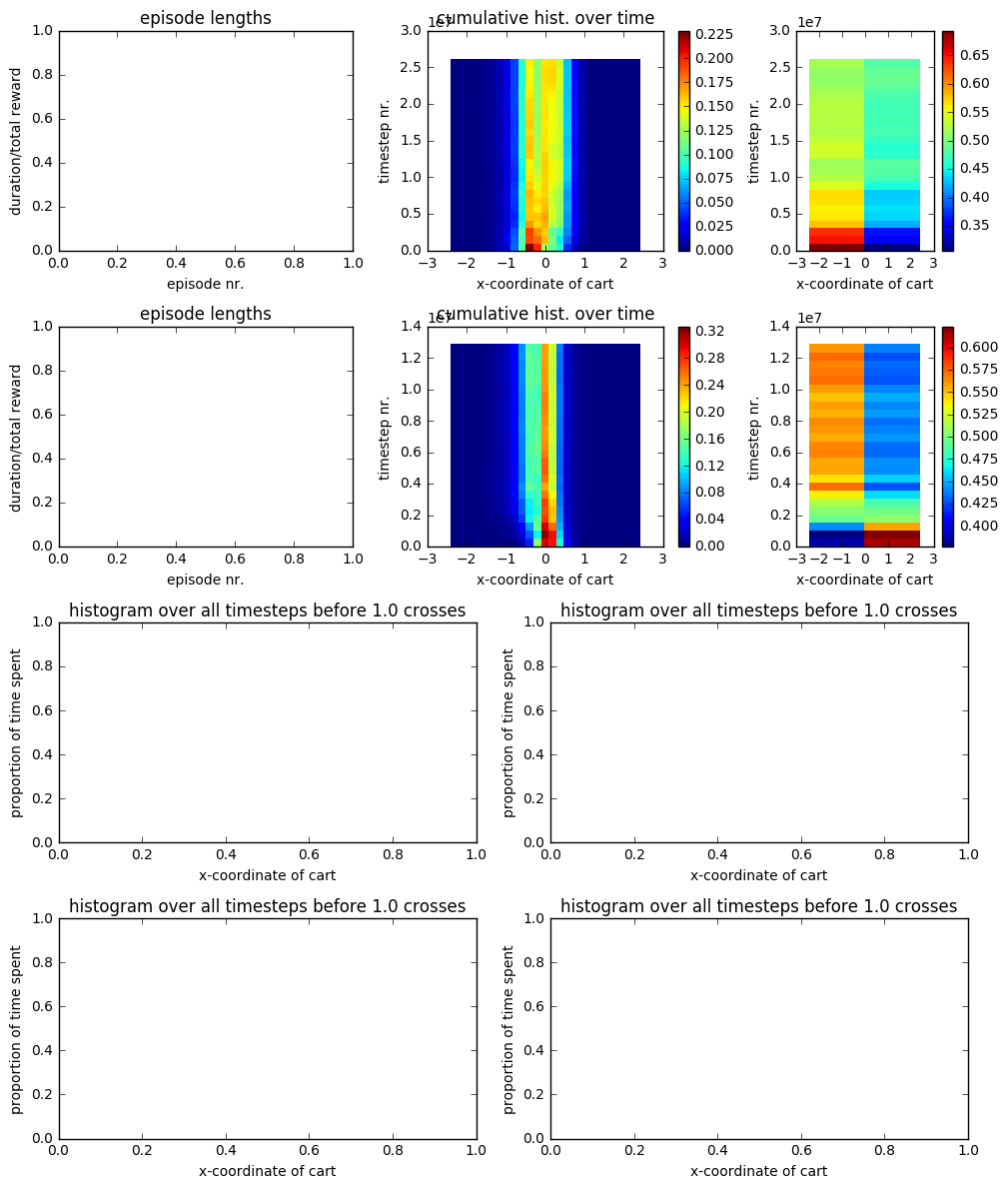In :
se.plot_xs_hist(interruptibility.mask_after_cross(xs).compressed(), ax, label='uninterrupted')

In :
with saveloaddata.load_res('Sarsa-rand-tiebreak', 'interrupted', data_dir_p) as res:

In :


In [ ]:
ax.legend()

In :
fig

Out: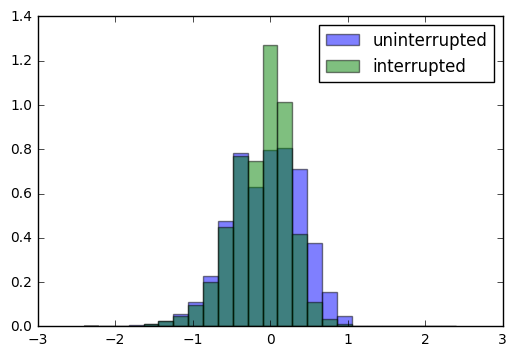In :
stats_experiments.plot_mean_std_change(before_cross_unint, label='uninterrupted')
stats_experiments.plot_mean_std_change(before_cross_int, label='interrupted')

In :
plt.legend()
plt.show()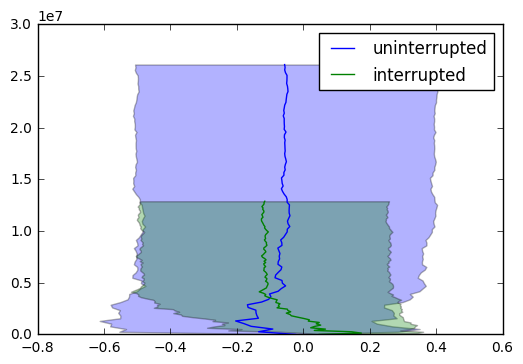In :
se.plot_jsd_devel(before_cross_unint)
plt.show()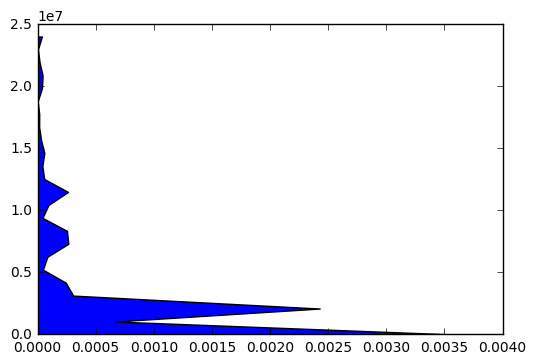In :
%debug

> /home/erle/.local/lib/python2.7/site-packages/matplotlib/axes/_axes.py(4771)fill_betweenx()
4769
4770         if not (y.shape == x1.shape == x2.shape == where.shape):
-> 4771             raise ValueError("Argument dimensions are incompatible")
4772

ipdb> y
masked_array(data = [0.0 1044651.76 2089303.52 3133955.2800000003 4178607.04 5223258.8
6267910.5600000005 7312562.32 8357214.08 9401865.84 10446517.6 11491169.36
12535821.120000001 13580472.88 14625124.64 15669776.4 16714428.16
17759079.92 18803731.68 19848383.44 20893035.2 21937686.96 22982338.72
24026990.48 25071642.240000002],
mask = [False False False False False False False False False False False False
False False False False False False False False False False False False
False],
fill_value = 1e+20)

ipdb> q

In :
fig, ax = plt.subplots()
ax.set_xscale('log')
se.plot_jsd_comp_final(before_cross_unint, ax=ax)

In :
ax.set_xlim([0.0, 0.03])

Out:
(0.0, 0.03)
In :
ax.get_xlim()

Out:
(1.0000000000000001e-05, 0.10000000000000001)
In :
plt.show()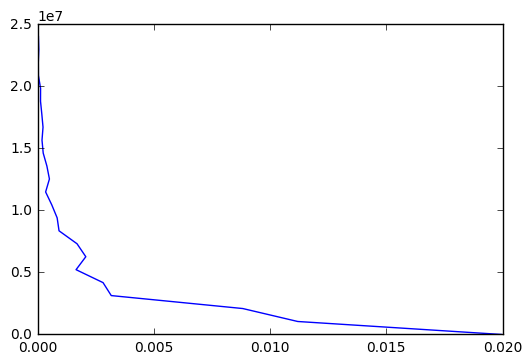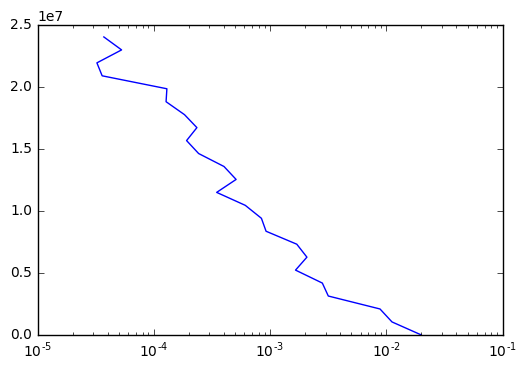Interesting: Interruptions don't move the whole thing to the left, but limit variance.

In :
fig, ax = plt.subplots()
mesh = stats_experiments.plot_xss_cum_hist_change(xs, ax, bins=25)
#plt.colorbar(mesh)
plt.show()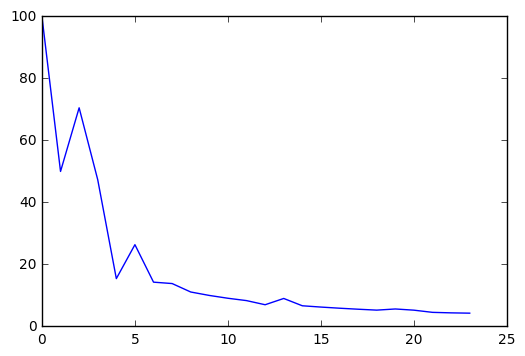In :
del xs

In :
p = '../data/Sarsa-disc-uninterrupted-xe-170221003432.pickle'
with open(p, 'rb') as f: